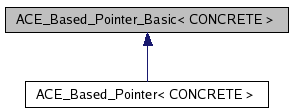# ACE_Based_Pointer_Basic< CONCRETE > Class Template Reference

A proxy that keeps track of the relative offset of a "pointer" from its base address. This class makes it possible to transparently use "pointers" in shared memory as easily as programming with pointers to local memory. In particular, we don't need to ensure that the base addresses of all the pointers are mapped into separate processes at the same absolute memory base address. More...

`#include <Based_Pointer_T.h>`

Inheritance diagram for ACE_Based_Pointer_Basic< CONCRETE >:[legend]

List of all members.

## Public Member Functions

ACE_Based_Pointer_Basic (void)
ACE_Based_Pointer_Basic (CONCRETE *initial)
ACE_Based_Pointer_Basic (const ACE_Based_Pointer_Basic< CONCRETE > &)
Copy constructor.
ACE_Based_Pointer_Basic (const void *base_addr, int o)
void operator= (CONCRETE *from)
Pseudo-assignment operator.
void operator= (const ACE_Based_Pointer_Basic< CONCRETE > &)
Pseudo-assignment operator.
CONCRETE & operator* (void) const
Dereference operator.
bool operator< (const ACE_Based_Pointer_Basic< CONCRETE > &) const
Less than operator.
bool operator<= (const ACE_Based_Pointer_Basic< CONCRETE > &) const
Less than or equal operator.
bool operator> (const ACE_Based_Pointer_Basic< CONCRETE > &) const
Greater than operator.
bool operator>= (const ACE_Based_Pointer_Basic< CONCRETE > &) const
Greater than or equal operator.
bool operator== (const ACE_Based_Pointer_Basic< CONCRETE > &) const
Equality operator.
bool operator!= (const ACE_Based_Pointer_Basic< CONCRETE > &) const
Inequality operator.
CONCRETE & operator[] (int index) const
Subscript operator.
void operator+= (int index)
Increment operator.
operator CONCRETE * () const
Returns the underlying memory address of the smart pointer.
CONCRETE * addr (void) const
Returns the underlying memory address of the smart pointer.
void dump (void) const
Dump the state of the object.

## Public Attributes

ACE_ALLOC_HOOK_DECLARE
Declare the dynamic allocation hooks.

## Protected Attributes

ptrdiff_t target_
ptrdiff_t base_offset_
Keep track of our offset from the base pointer.

## Detailed Description

### template<class CONCRETE> class ACE_Based_Pointer_Basic< CONCRETE >

A proxy that keeps track of the relative offset of a "pointer" from its base address. This class makes it possible to transparently use "pointers" in shared memory as easily as programming with pointers to local memory. In particular, we don't need to ensure that the base addresses of all the pointers are mapped into separate processes at the same absolute memory base address.

## Constructor & Destructor Documentation

template<class CONCRETE>
 ACE_Based_Pointer_Basic< CONCRETE >::ACE_Based_Pointer_Basic ( void ) ` [inline]`

This constructor initializes the <base_offset_> by asking the <ACE_BASED_POINTER_REPOSITORY> Singleton for the base address of the memory region within which it is instantiated. Two results are possible:

1. An <ACE_*_Memory_Pool> has stored a base address/size pair and the new based-pointer instance is located between the base address and the base address + size - 1. In this case, the repository returns the base address.

2. No suitable address/size pair was found. The repository assumes an address in the regular (not mapped) virtual address space of the process and returns 0. In this case, the based-pointer uses its address as an offset to it's base address 0.

template<class CONCRETE>
 ACE_Based_Pointer_Basic< CONCRETE >::ACE_Based_Pointer_Basic ( CONCRETE * initial ) ` [inline]`

Initialize this object using the initial pointer. This constructor initializes the <base_offset_> by asking the <ACE_BASED_POINTER_REPOSITORY> Singleton for the base address of the memory region within which it is instantiated. Three results are possible:

1. An <ACE_*_Memory_Pool> has stored a base address/size pair and the new based-pointer instance is located between the base address and the base address + size - 1. In this case, the repository returns the base address.

2. No suitable address/size pair was found. The repository assumes an address in the regular (not mapped) virtual address space of the process and returns 0. In this case, the based-pointer uses its address as an offset to its base address 0.

3. If initial is 0 then set the value of <target_> to -1, which indicates a "NULL" pointer.

template<class CONCRETE>
 ACE_Based_Pointer_Basic< CONCRETE >::ACE_Based_Pointer_Basic ( const ACE_Based_Pointer_Basic< CONCRETE > & ) ` [inline]`

Copy constructor.

template<class CONCRETE>
 ACE_Based_Pointer_Basic< CONCRETE >::ACE_Based_Pointer_Basic ( const void * base_addr, int o ) ` [inline]`

Constructor for know base address. o is only used to resolve overload ambiguity.

## Member Function Documentation

template<class CONCRETE>
 ACE_INLINE void ACE_Based_Pointer_Basic< CONCRETE >::operator= ( CONCRETE * from ) ` [inline]`

Pseudo-assignment operator.

Reimplemented in ACE_Based_Pointer< CONCRETE >.

template<class CONCRETE>
 ACE_INLINE void ACE_Based_Pointer_Basic< CONCRETE >::operator= ( const ACE_Based_Pointer_Basic< CONCRETE > & rhs ) ` [inline]`

Pseudo-assignment operator.

template<class CONCRETE>
 ACE_INLINE CONCRETE & ACE_Based_Pointer_Basic< CONCRETE >::operator* ( void ) const` [inline]`

Dereference operator.

template<class CONCRETE>
 ACE_INLINE bool ACE_Based_Pointer_Basic< CONCRETE >::operator< ( const ACE_Based_Pointer_Basic< CONCRETE > & rhs ) const` [inline]`

Less than operator.

template<class CONCRETE>
 ACE_INLINE bool ACE_Based_Pointer_Basic< CONCRETE >::operator<= ( const ACE_Based_Pointer_Basic< CONCRETE > & rhs ) const` [inline]`

Less than or equal operator.

template<class CONCRETE>
 ACE_INLINE bool ACE_Based_Pointer_Basic< CONCRETE >::operator> ( const ACE_Based_Pointer_Basic< CONCRETE > & rhs ) const` [inline]`

Greater than operator.

template<class CONCRETE>
 ACE_INLINE bool ACE_Based_Pointer_Basic< CONCRETE >::operator>= ( const ACE_Based_Pointer_Basic< CONCRETE > & rhs ) const` [inline]`

Greater than or equal operator.

template<class CONCRETE>
 ACE_INLINE bool ACE_Based_Pointer_Basic< CONCRETE >::operator== ( const ACE_Based_Pointer_Basic< CONCRETE > & rhs ) const` [inline]`

Equality operator.

template<class CONCRETE>
 ACE_INLINE bool ACE_Based_Pointer_Basic< CONCRETE >::operator!= ( const ACE_Based_Pointer_Basic< CONCRETE > & rhs ) const` [inline]`

Inequality operator.

template<class CONCRETE>
 ACE_INLINE CONCRETE & ACE_Based_Pointer_Basic< CONCRETE >::operator[] ( int index ) const` [inline]`

Subscript operator.

template<class CONCRETE>
 ACE_INLINE void ACE_Based_Pointer_Basic< CONCRETE >::operator+= ( int index ) ` [inline]`

Increment operator.

template<class CONCRETE>
 ACE_INLINE ACE_Based_Pointer_Basic< CONCRETE >::operator CONCRETE * ( ) const` [inline]`

Returns the underlying memory address of the smart pointer.

template<class CONCRETE>
 ACE_INLINE CONCRETE * ACE_Based_Pointer_Basic< CONCRETE >::addr ( void ) const` [inline]`

Returns the underlying memory address of the smart pointer.

template<class CONCRETE>
 void ACE_Based_Pointer_Basic< CONCRETE >::dump ( void ) const` [inline]`

Dump the state of the object.

## Member Data Documentation

template<class CONCRETE>
 ACE_Based_Pointer_Basic< CONCRETE >::ACE_ALLOC_HOOK_DECLARE

Declare the dynamic allocation hooks.

template<class CONCRETE>
 ptrdiff_t ACE_Based_Pointer_Basic< CONCRETE >::target_` [protected]`

template<class CONCRETE>
 ptrdiff_t ACE_Based_Pointer_Basic< CONCRETE >::base_offset_` [protected]`

Keep track of our offset from the base pointer.

The documentation for this class was generated from the following files:

Generated on Wed Apr 23 02:38:09 2008 for ACE by1.5.5